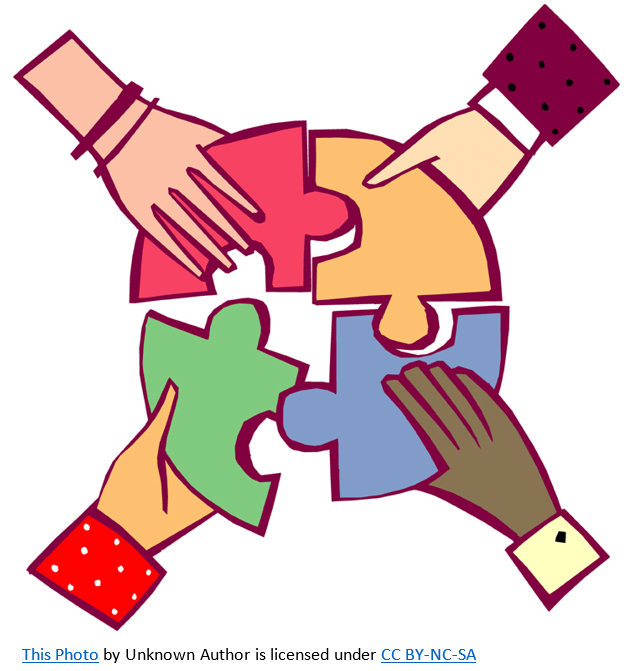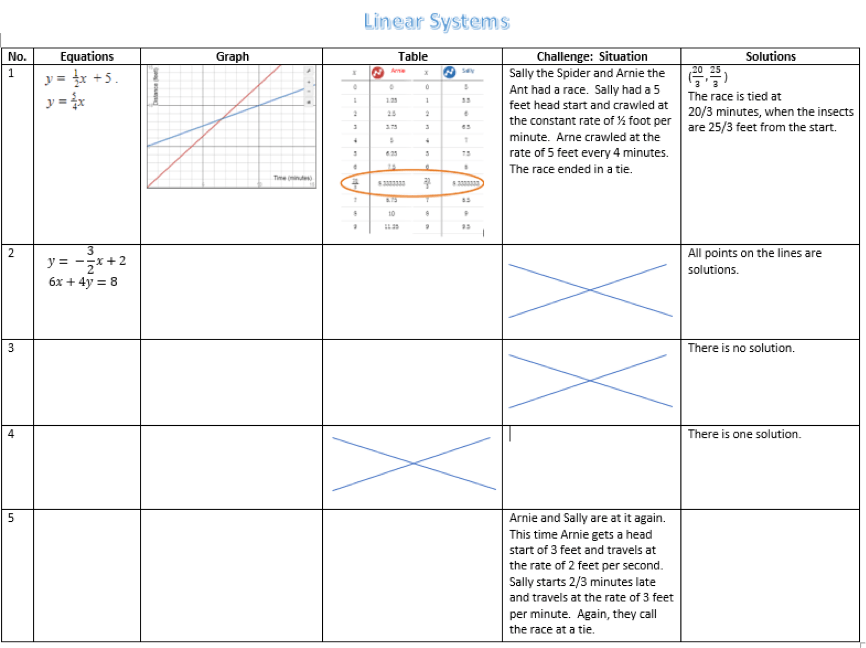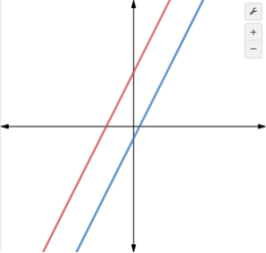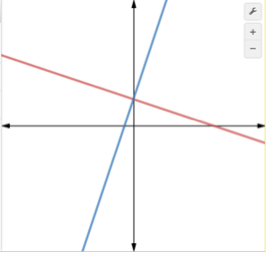# LINR 3 | Lesson 1 | Making ConnectionsBelow is information about 5 linear systems represented in different ways. The first is the Sally/Arnie race. Fill-out the table for the next four (you are encouraged to use Desmos.com). Show how you solved the problem using each representation. Student Worksheet (Your work should also show connections between representations.)Solutions to linear systems can be shown for each representation as follows:

 Equations Substitution (or linear combinations – not highlighted in this module) Graphs Intersection of lines (exact solution not always possible) Table Same points in both tables (exact solution not always possible/often very tedious to find) Situation Examination (exact solution not always possible)

Three situations

 ParallelIntersectingCoincident$$y=2x+4$$ $$y=2x-1$$ Soving… $$2x+4=2x-1$$ $$4\neq-1$$ (never true, no matter the value of $$x$$) $$y=-\Large \frac{1}{3}\normalsize x+2$$ $$y=3x+2$$ Solving… $$-\Large \frac{1}{3}\normalsize x+2=3x+2$$ $$0=\Large \frac{10}{3} \normalsize x$$ $$x=0$$ $$y=2$$ $$(0,2)$$ (there is only one $$(x,y)$$ point of intersection) $$y=2x+4$$ $$4x-2y=-8$$ Solving… $$4x-2(2x+4)=-8$$ $$4x-4x-8=-8$$ $$-8=-8$$ (no matter the value of $$x$$, always true) No solutions One solution Infinite solutions